# Maths - Sheaf

The sheaf structure is a powerful idea that goes across many mathematical subjects and therefore it can be approached from different directions. The concept arose from topology and the concept of an open set, this is discussed further down the page.

### Fibre With Structure

The page about fibre bundles here shows how reversing a map between sets gives some interesting structures. Here we extend that idea.

The diagram on the right is intended to show a morphism between partial orders. So the blue arrows represent ≥ and the arrow 'ab' means b ≥ a.

The red arrows show the morphism between the partial orders and these all need to play nicely together.So how can this morphism be reversed?

The diagram on the right is the best I can do although somehow it doesn't seem completely correct, for instance it seems to imply that c ≥ a whereas the original diagram above doesn't imply that.

In order to be consistent this morphism needs to be a local homeomorphism which is a function between topological spaces that preserves local but not necessarily global structure.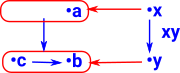### Local and Global Structures

The interesting thing is that this is dividing the structure into 'local' structure and 'global' structure.

It seems natural to have morphisms that preserve global structure but may compress local structure. This does give a morphism that is reversible and we get a fibre bundle with some extra structure.

However, that's not what we want for a sheaf, what we want is a morphism that preserves local structure but may compress global structure.

### Local Homeomorphism

In order to be reversible this morphism needs to be a local homeomorphism, as in the example on the right, here the local structure is preserved.Reversing this morphism gives a fibre structure, as described on this page, but with additional structure. Now the local structure inside the xy type (on the right of the diagram) maps to the same structure between the family of types.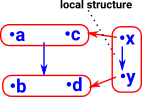Here I have just rotated the above diagram by 90 degrees just to show in the same way we did with fibres.

So each element x,y of x->y indexes a type.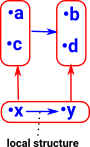## Section

If we have a subset (subtype?) of the indexing type then this translates to a subset of the type family.So far we have only described only part of what a sheaf is. What is described so far is a presheaf, this gives us lots of copies of the local structure. Making a presheaf into a sheaf allows us to glue these local structures together to form a more interesting structure.

## Presheaf in Category Theory

A 'presheaf' category is a special case of a functor category (see page here). It is a contravarient functor from a category 'C' to Set.

Since it is contravarient it is usually written:

CopSet

or

SetCop

There is more about presheaves on the page here.

### Presheaf Example - Single Element Set

A very simple example would be where Cop is a single element set (terminal object in set).

Hom( Cop, Set) therefore contains set of single arrows, one for every element of the set.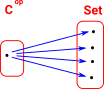### Presheaf Example - Graph

Here Cop is a category with two objects E (for edge) and V (for vertex) also two arrows s (for source) and t (for target).

This allows us to build a structure on top of set where the diagram on the right commutes.

We can therefore build up complex graphs from individual vertices and edges.### Presheaf Example - Relational Database

Here Cop is a database schema.

This imposes a structure on the sets which are the database tables.

This implements a category of simplical databases.## Presheaf on a Topological Space

So far we have not yet discussed a sheaf on a topological space, this is where the concept originated and adds more interesting ideas.

We can get to this concept by replacing the sets discussed above with 'open sets' (more about open sets on page here).

We can have a presheaf of any structure 'A' on a topological space 'X'.

A presheaf of sets 'A' on 'X' is valid but it does not give much structure to relate the open sets. Something with an abelian group structure for example (such as vectors) gives us more structure to work with. More about open sets on page here.

 A presheaf of abelian group 'A' on 'X' assigns to each open set UX an abelian group A(U). and that assigns to each pair UV of open sets a homomorphism called the restriction rU,V : A(V) -> A(U)in such a way that rU,U = 1 (identity map)rU,VrV,W = rU,W (composition)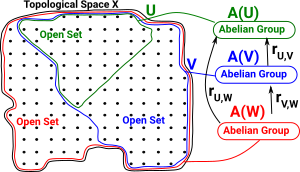## Presheaf in Category Theory

In category theory terms:

• X is a topological space
• A presheaf of abelian groups on X is a contravariant functor from the category of open subsets of X and inclusions to the category of abelian groups.
 Let C=Top(X) the category whose objects are the open subsets of X. C can be represented as a poset of open sets in a topological space with the morphisms being inclusion maps. There is a single arrow from V to U if V is included in U or V=U (V is a subset of U). Hom(V,U) has one element. If V is not a subset of U there is no arrow. Hom(V,U) is empty. There is never more than one arrow between any two open sets, if it exists the arrow is unique. In the same way as for fibres we usually reverse the arrow so that it is a contravarient arrow from Cop to Set: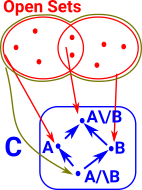A presheaf on a category C is a functor F : Cop-> Set

For instance a presheaf can be a contravariant functor from the category Top(X) to the category Ab of Abelian groups (which may also have more structure).

Sheaves are discussed from a category theory point of view on the page here.

## Developing Fibre Bundle Approach

The page here introduced fibre bundles.

For example, where there is a family of types indexed by elements of another type.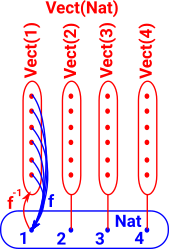The type families in fibre bundles are disjoint. One way to extend that concept is to allow an overlapping type family.open sets simplectical complex In some cases we can convert between open sets and simplices. see Wikipedia articles:### Sheaf and Co-Sheaf

Allow us to translate between physical sources of data and open sets or simpicies.

sheaf Co-sheaf
vertex weighted hyperedge weighted
vertex has non-trvial stalk. toplex has non-trvial stalk.
All restrictions are zero maps All extensions are zero maps
The resulting sheaf is flabby The resulting cosheaf is coflabby

### Sheaf

A sheaf is a presheaf that satisfies the following two additional axioms:

• (Locality) If U is an open covering of an open set U , and if s , tF(U) have the property s | U i = t | U i for each set Ui of the covering, then s = t and
• (Gluing) If U is an open covering of an open set U, and if for each iI a section si is given such that for each pair Ui ,Uj of the covering sets the restrictions of si and sj agree on the overlaps, so s i | U i ∩ U j = s j | U i ∩ U j, then there is a section sF ( U ) such that s | U i = sifor each iI.

Where the notation used is:

• s | U i means s restricted to U i
• s is a section of the space over U

more notation see box on right.

### Diagrams

 We can think of this in a combinatorics way. The relations are shown more clearly in an attachment diagram here. This shows subset relations with the arrows going from lower dimension to higher dimension.A sheaf assigns some data spaces to the attachment diagram above. In this case reals (ℜ) - A sheaf of vector spaces. Each such set is called a stalk over the simplex. ℜ in all the points gives a vector ℜ² a matrix links the vectors, each such function is known as a restriction.Section - an assignment of values from each of the stalks that is consistent with the restrictions.

Sections can be:

• Global - defined everywhere.
• Local - defined for some part.

If all local sections extend to global sections the sheaf is called flabby/flasque (don't have interesting invariant's).

 metadata block see also: Michael Robinson - Youtube from two-day short course on Applied Sheaf Theory: Lecture 1 Lecture 2 - about simplicies & topology (sound on video is not good - quiet and delayed) Lecture 3 Lecture 4 Lecture 5 Lecture 6 Lecture 7 Lecture 8 Correspondence about this page Book Shop - Further reading . Where I can, I have put links to Amazon for books that are relevant to the subject, click on the appropriate country flag to get more details of the book or to buy it from them.Introduction to Topological Manifolds (Graduate Texts in Mathematics S.) Other Books about Curves and Surfaces

This site may have errors. Don't use for critical systems.